Скачать презентацию FIN 437 Vicentiu Covrig Stocks and their valuation

303085f94372ab2c5f8daeb036868b91.ppt

• Количество слайдов: 16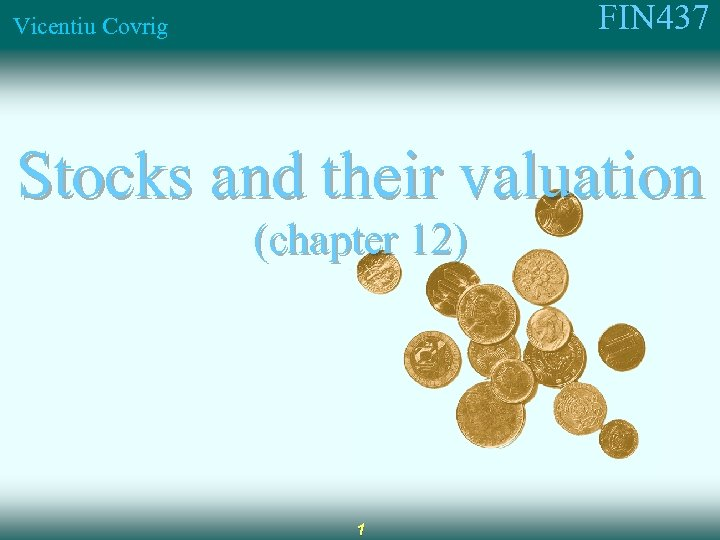FIN 437 Vicentiu Covrig Stocks and their valuation (chapter 12) 1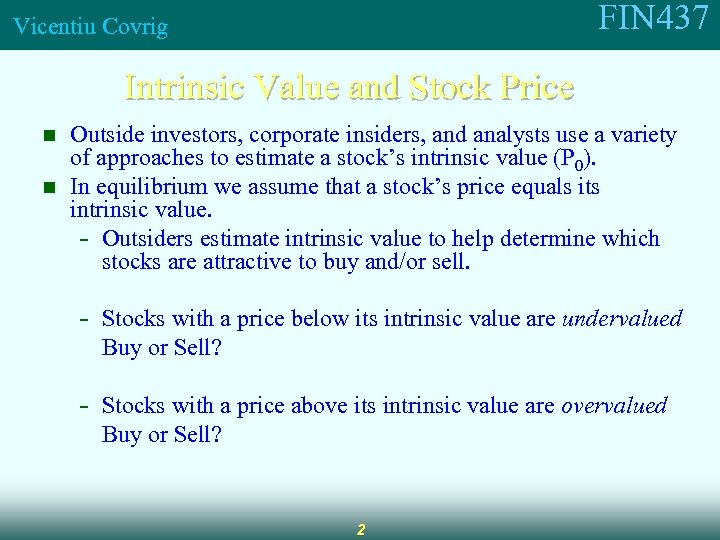FIN 437 Vicentiu Covrig Intrinsic Value and Stock Price n n Outside investors, corporate insiders, and analysts use a variety of approaches to estimate a stock’s intrinsic value (P 0). In equilibrium we assume that a stock’s price equals its intrinsic value. - Outsiders estimate intrinsic value to help determine which stocks are attractive to buy and/or sell. - Stocks with a price below its intrinsic value are undervalued Buy or Sell? - Stocks with a price above its intrinsic value are overvalued Buy or Sell? 2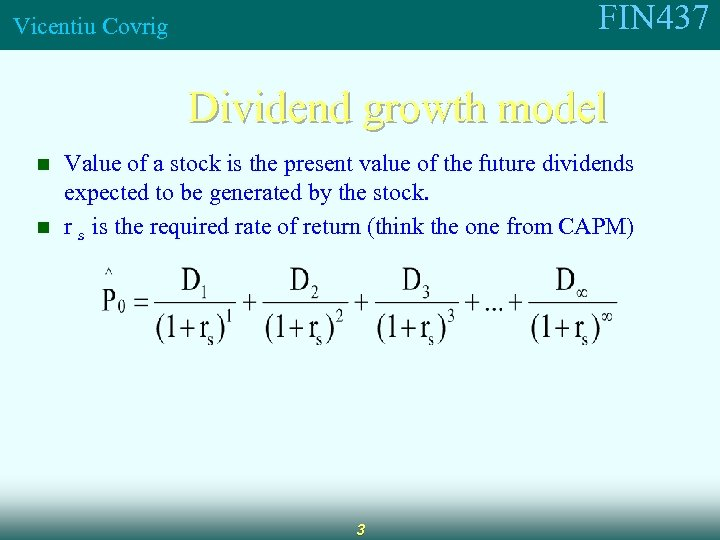FIN 437 Vicentiu Covrig Dividend growth model n n Value of a stock is the present value of the future dividends expected to be generated by the stock. r s is the required rate of return (think the one from CAPM) 3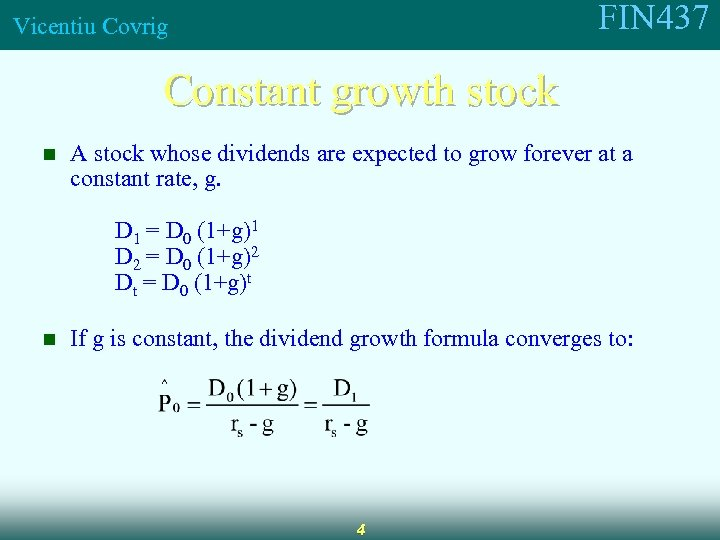FIN 437 Vicentiu Covrig Constant growth stock n A stock whose dividends are expected to grow forever at a constant rate, g. D 1 = D 0 (1+g)1 D 2 = D 0 (1+g)2 Dt = D 0 (1+g)t n If g is constant, the dividend growth formula converges to: 4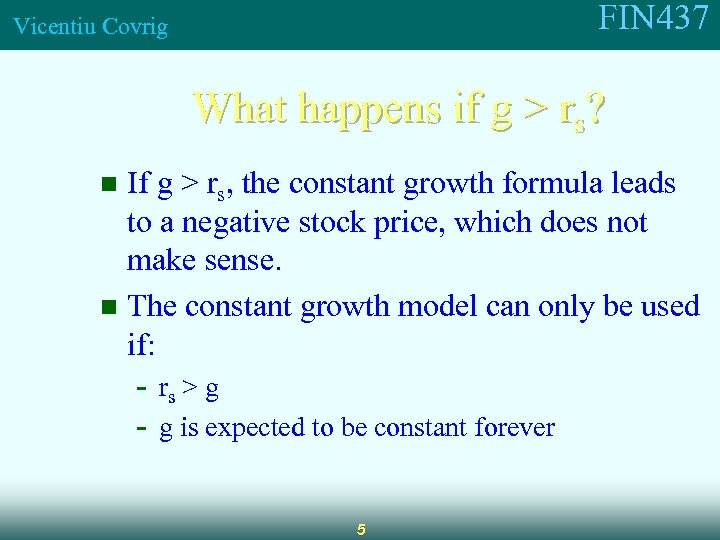FIN 437 Vicentiu Covrig What happens if g > rs? If g > rs, the constant growth formula leads to a negative stock price, which does not make sense. n The constant growth model can only be used if: n - rs > g - g is expected to be constant forever 5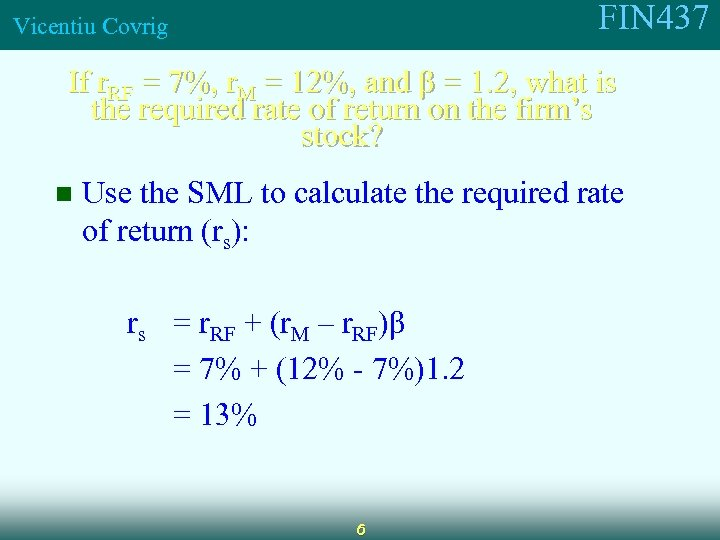FIN 437 Vicentiu Covrig If r. RF = 7%, r. M = 12%, and β = 1. 2, what is the required rate of return on the firm’s stock? n Use the SML to calculate the required rate of return (rs): rs = r. RF + (r. M – r. RF)β = 7% + (12% - 7%)1. 2 = 13% 6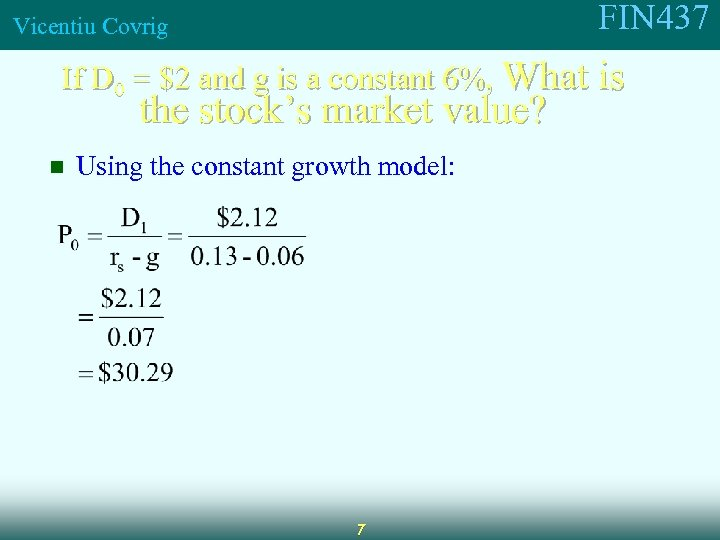FIN 437 Vicentiu Covrig If D 0 = \$2 and g is a constant 6%, What is the stock’s market value? n Using the constant growth model: 7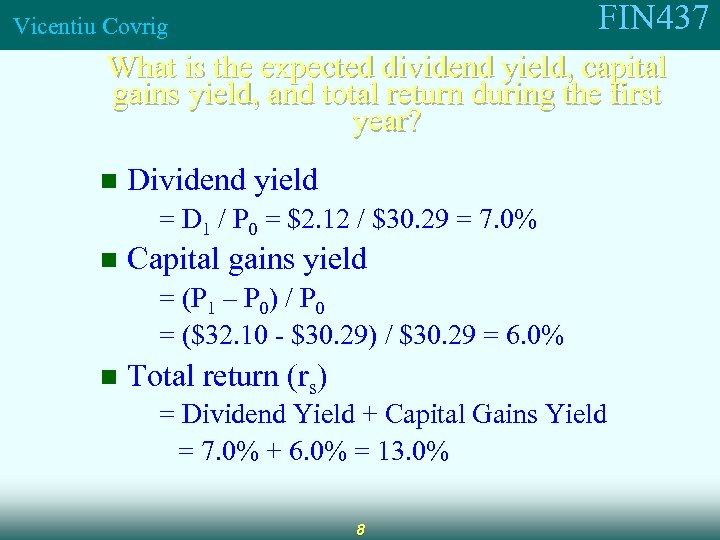FIN 437 Vicentiu Covrig What is the expected dividend yield, capital gains yield, and total return during the first year? n Dividend yield = D 1 / P 0 = \$2. 12 / \$30. 29 = 7. 0% n Capital gains yield = (P 1 – P 0) / P 0 = (\$32. 10 - \$30. 29) / \$30. 29 = 6. 0% n Total return (rs) = Dividend Yield + Capital Gains Yield = 7. 0% + 6. 0% = 13. 0% 8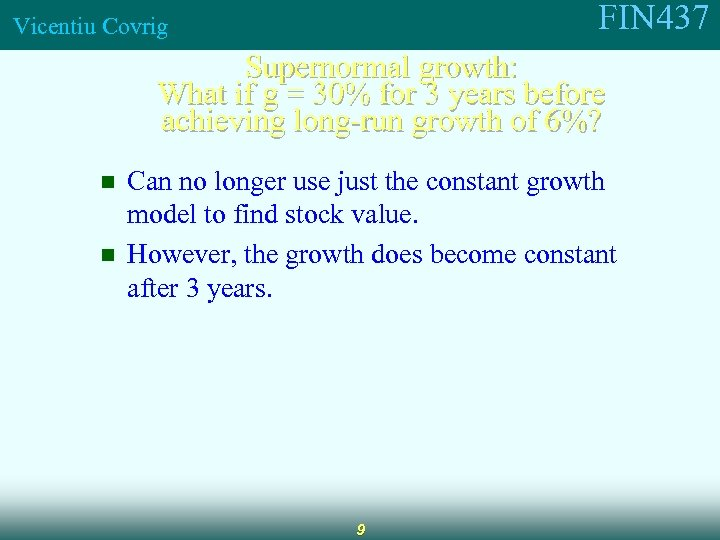FIN 437 Vicentiu Covrig Supernormal growth: What if g = 30% for 3 years before achieving long-run growth of 6%? n n Can no longer use just the constant growth model to find stock value. However, the growth does become constant after 3 years. 9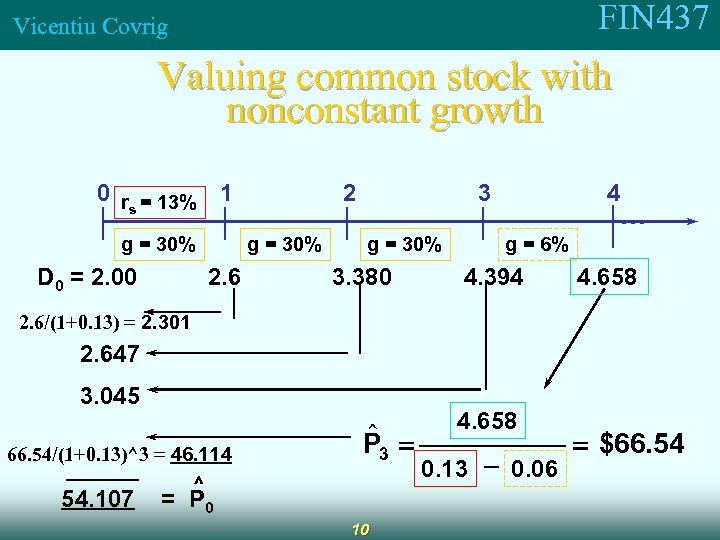FIN 437 Vicentiu Covrig Valuing common stock with nonconstant growth 0 r = 13% 1 s g = 30% D 0 = 2. 00 2 g = 30% 2. 6 3 g = 30% 3. 380 4 . . . g = 6% 4. 394 4. 658 2. 6/(1+0. 13) = 2. 301 2. 647 3. 045 66. 54/(1+0. 13)^3 = 46. 114 54. 107 \$ P 3 = ^ = P 0 10 4. 658 0. 13 - 0. 06 = \$66. 54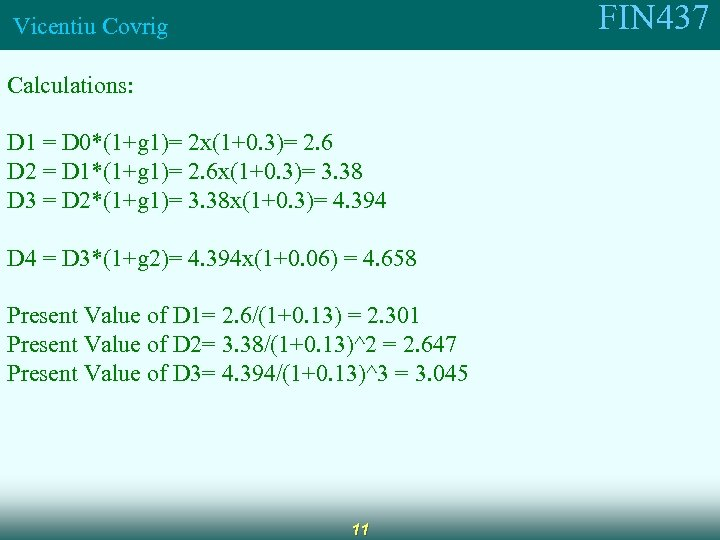FIN 437 Vicentiu Covrig Calculations: D 1 = D 0*(1+g 1)= 2 x(1+0. 3)= 2. 6 D 2 = D 1*(1+g 1)= 2. 6 x(1+0. 3)= 3. 38 D 3 = D 2*(1+g 1)= 3. 38 x(1+0. 3)= 4. 394 D 4 = D 3*(1+g 2)= 4. 394 x(1+0. 06) = 4. 658 Present Value of D 1= 2. 6/(1+0. 13) = 2. 301 Present Value of D 2= 3. 38/(1+0. 13)^2 = 2. 647 Present Value of D 3= 4. 394/(1+0. 13)^3 = 3. 045 11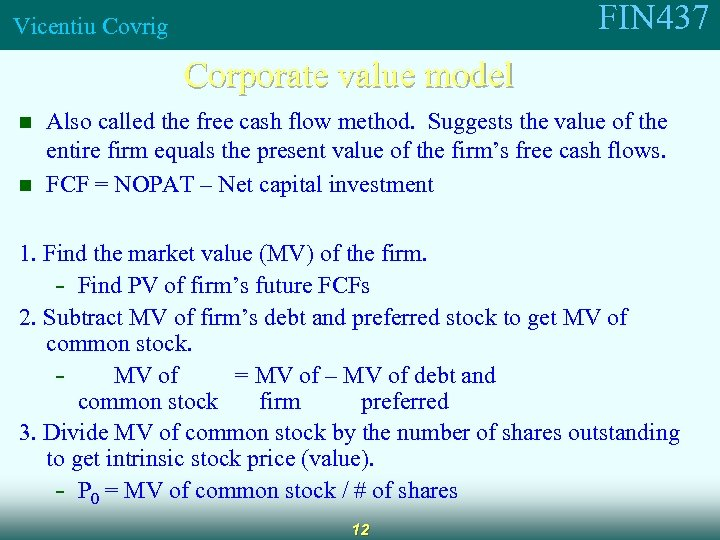FIN 437 Vicentiu Covrig Corporate value model n n Also called the free cash flow method. Suggests the value of the entire firm equals the present value of the firm’s free cash flows. FCF = NOPAT – Net capital investment 1. Find the market value (MV) of the firm. - Find PV of firm’s future FCFs 2. Subtract MV of firm’s debt and preferred stock to get MV of common stock. MV of = MV of – MV of debt and common stock firm preferred 3. Divide MV of common stock by the number of shares outstanding to get intrinsic stock price (value). - P 0 = MV of common stock / # of shares 12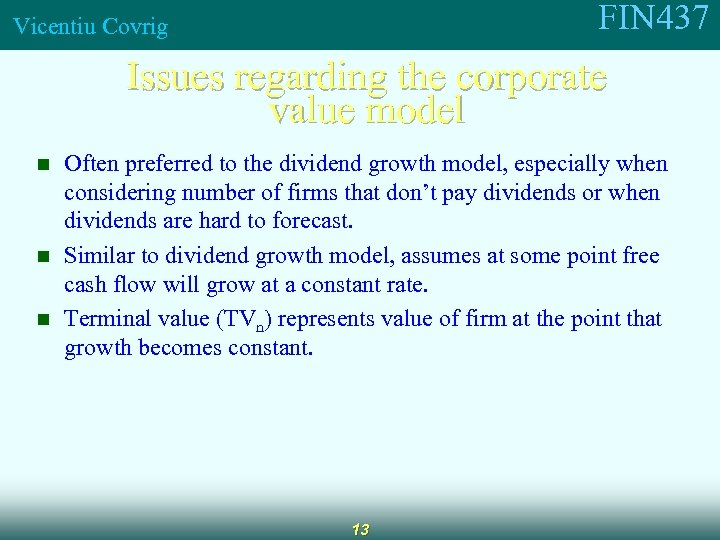FIN 437 Vicentiu Covrig Issues regarding the corporate value model n n n Often preferred to the dividend growth model, especially when considering number of firms that don’t pay dividends or when dividends are hard to forecast. Similar to dividend growth model, assumes at some point free cash flow will grow at a constant rate. Terminal value (TVn) represents value of firm at the point that growth becomes constant. 13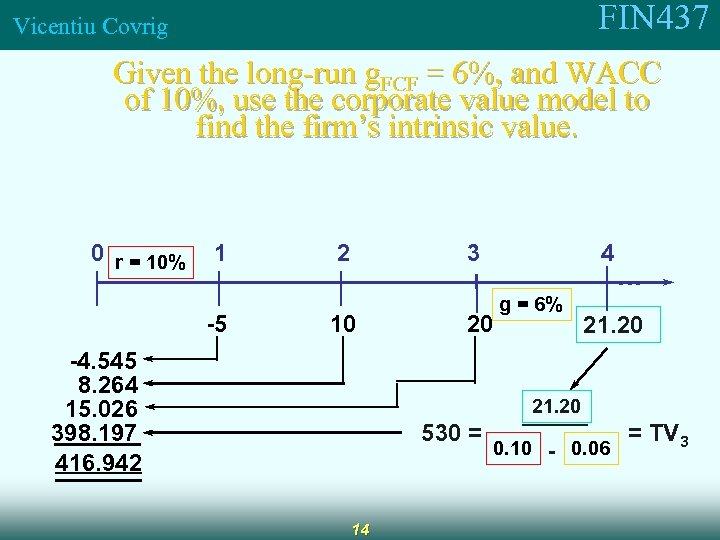FIN 437 Vicentiu Covrig Given the long-run g. FCF = 6%, and WACC of 10%, use the corporate value model to find the firm’s intrinsic value. 0 r = 10% 1 -5 2 3 10 -4. 545 8. 264 15. 026 398. 197 416. 942 4 20 g = 6% . . . 21. 20 530 = 14 0. 10 - 0. 06 = TV 3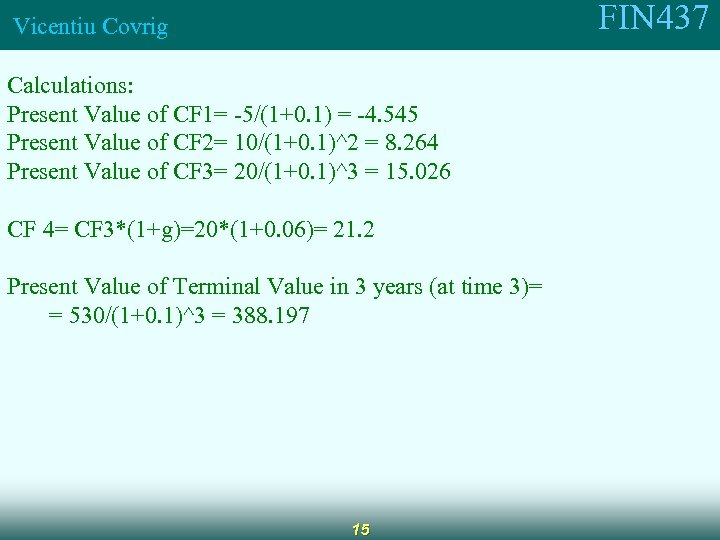FIN 437 Vicentiu Covrig Calculations: Present Value of CF 1= -5/(1+0. 1) = -4. 545 Present Value of CF 2= 10/(1+0. 1)^2 = 8. 264 Present Value of CF 3= 20/(1+0. 1)^3 = 15. 026 CF 4= CF 3*(1+g)=20*(1+0. 06)= 21. 2 Present Value of Terminal Value in 3 years (at time 3)= = 530/(1+0. 1)^3 = 388. 197 15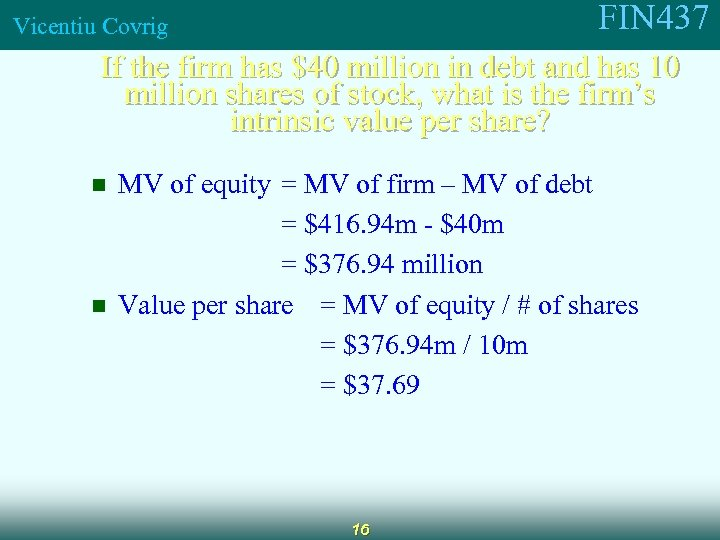FIN 437 Vicentiu Covrig If the firm has \$40 million in debt and has 10 million shares of stock, what is the firm’s intrinsic value per share? n n MV of equity = MV of firm – MV of debt = \$416. 94 m - \$40 m = \$376. 94 million Value per share = MV of equity / # of shares = \$376. 94 m / 10 m = \$37. 69 16